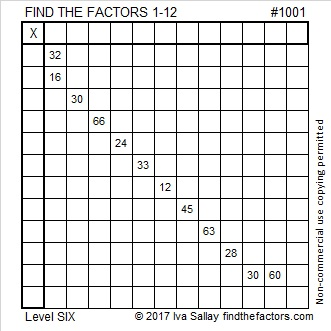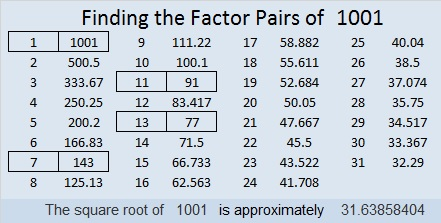# 1001 and Level 6

There is only one multiplication table that has the numbers you see in this puzzle exactly where you see them here. Can you find the factors that create that multiplication table? This is a level 6 puzzle so it won’t be easy, but it can be done by just using logic and the basic 1-12 multiplication facts.Print the puzzles or type the solution in this excel file: 12 factors 993-1001

1001 is the product of three consecutive prime numbers:
7 × 11 × 13 = 1001

1001 is also the sum of fifteen consecutive prime numbers:
37 + 41 + 43 + 47 + 53 + 59 + 61 + 67 + 71 + 73 + 79 + 83 + 89 + 97 + 101 = 1001

1001 is the 26th pentagonal number.

1001 is the hypotenuse of a Pythagorean triple:
385² + 924² = 1001²

1001 is a palindrome in base 10 and in base 25:
It’s 1F1 in BASE 25 (F is 15 base 10) because 1(625) + 15(25) + 1(1) = 1001

• 1001 is a composite number.
• Prime factorization: 1001 = 7 × 11 × 13
• The exponents in the prime factorization are 1, 1, and 1. Adding one to each and multiplying we get (1 + 1)(1 + 1)(1 + 1) = 2 × 2 × 2 = 8. Therefore 1001 has exactly 8 factors.
• Factors of 1001: 1, 7, 11, 13, 77, 91, 143, 1001
• Factor pairs: 1001 = 1 × 1001, 7 × 143, 11 × 91, or 13 × 77
• 1001 has no square factors that allow its square root to be simplified. √1001 ≈ 31.63858This site uses Akismet to reduce spam. Learn how your comment data is processed.